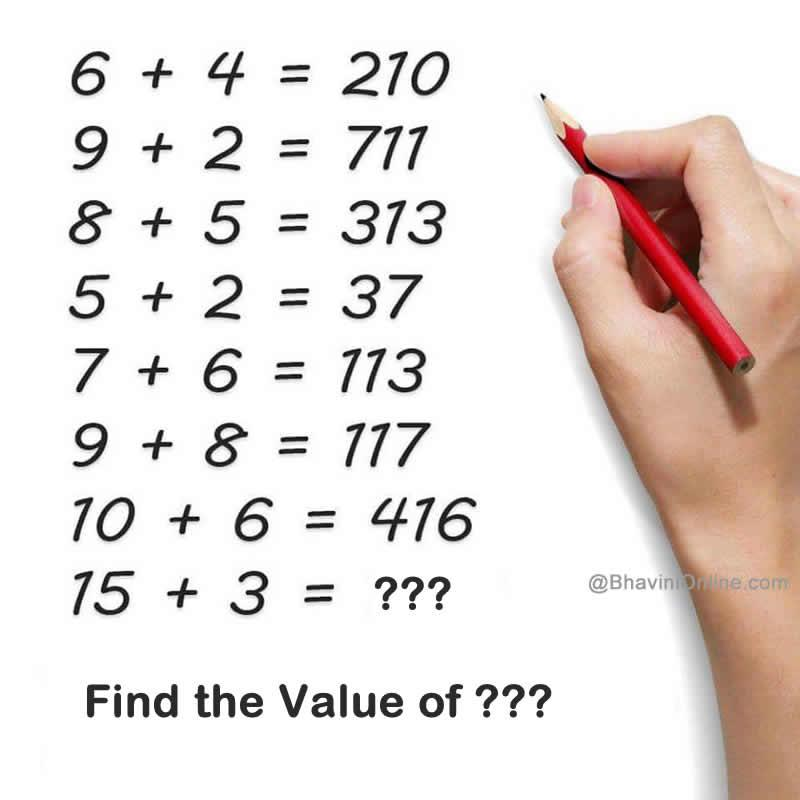# What Comes Next In This Sequence: If 6 + 4 = 210; 15 + 3 = ??

What comes next in this sequence;

6 + 4 = 210

9 + 2 = 711

8 + 5 = 313

5 + 2 = 37

7 + 6 = 113

9 + 8 = 117

10 + 6 = 416

15 + 3 = ???So were you able to solve the riddle? Leave your answers in the comment section below.